Background

Physically Based Rendering is not a technique, but a collection of graphics techniques aiming to achieve results as close as possible to the physical world. This means that these techniques are highly based on real world physics light behaviour formulas. However, in order for these techiniques to be plausible to be rendered in real-time, some approximations need to be made (that is where the "based" in PBR comes from!).

In this post I will talk about the PBR techniques I implemented in my Custom Engine (physically based shading, image based lighting, and atmospheric scattering) and what I learned while doing it. Lets go!

Physically Based Rendering

Introduction

My implementation is highly based on this tutorial. Its basis are the following:

• Be based on the microfacet surface model: this theory describes how at a microscopic level, all surfaces have a certain degree of roughness which will scatter light rays in a more or less chaotic way, depending on this roughness degree.• Be energy conserving: This means that light leaving a surface, can never be brighter than the light that fell upon it; so the sum of the diffuse and reflected light cannot be bigger than the received light. As you can see in the image, as the surface gets smoother, the light reflected takes over the diffuse color of the sphere.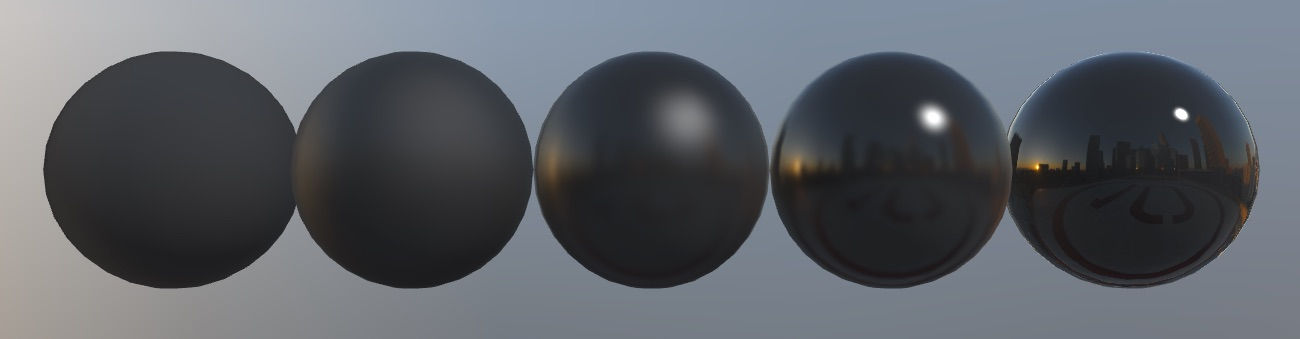• Use a physically based BRDF: The bidirectional reflectance distribution function used in this case is the Cook-Torrance BRDF. It is a function that models light behaviour in two different ways, making a difference between diffuse and specular reflection. This defines the way that light is reflected on the different surface models, and how the viewer sees the object.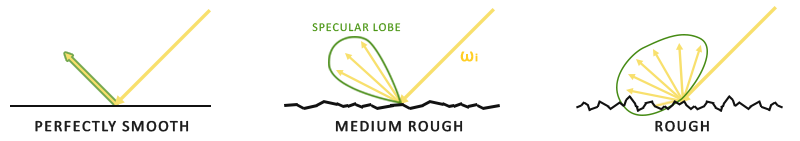In the end, we get the Cook-Torrance reflectance equation, a more specialized version of the rendering equation.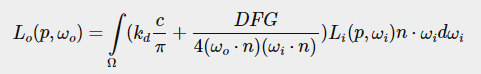I will not go into detail on how the reflectance function is translated into code, as the author of the post does a great job at explaining this. Once this functions are in the shader, computation for directional, point and spot lights is done in the shader, with the addition of the different light properties such as intensity/attenuation, ambient factor, color, cone angle in case of spot lights, and calculate the resulting light output keeping in mind the energy conservation rule.

refractedLight = 1.0 - reflectedLight

Now the lighting just needs the shadows to be applied to it, if any. Doing this is as simple as getting the resulting factor of shadow calculation and multiplying it by the output light.

This process is repeated for each light object, and summed to the final output value (as light is additive).

Image Based Lighting

For the IBL part, three preprocess passes are performed at the beggining of the application, in order to achieve the textures needed for the environment lighting of the PBR materials:

• Diffuse Irradiance: This process maps to a cube a blurred version of the environment cubemap, by convoluting each sample direction of the cubemap, and accumulating all other sample directions over a hemisphere, representing the sum of all indirect diffuse light of the scene hitting some surface with a certain normal direction. This texture result is known as irradiance map.• Pre-filtered environment map: This process is similar to the diffuse irradiance, but taking in roughness into account. Multiple level-of-detail cubemaps are convoluted, with more scattered sample vectors at each level (creating a blurrier result).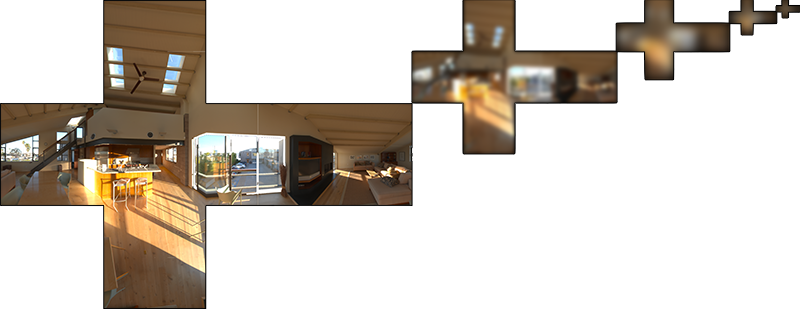• BRDF: The final bit that needs to be done is calculating the BRDF and mapping it to a lookup texture. Red represents a scale value, and green represents a bias value to the surface’s Fresnel1, while the horizontal and vertical texture coordinates represent the BRDF’s input n ⋅ ωi value and the input roughness value, respectively.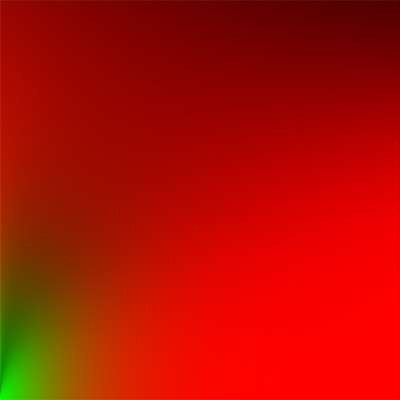Fresnel refers to the reflectivity occuring at different angles. Light landing on a surface at a grazing angle will be much more likely to reflect than that which hits a surface dead-on.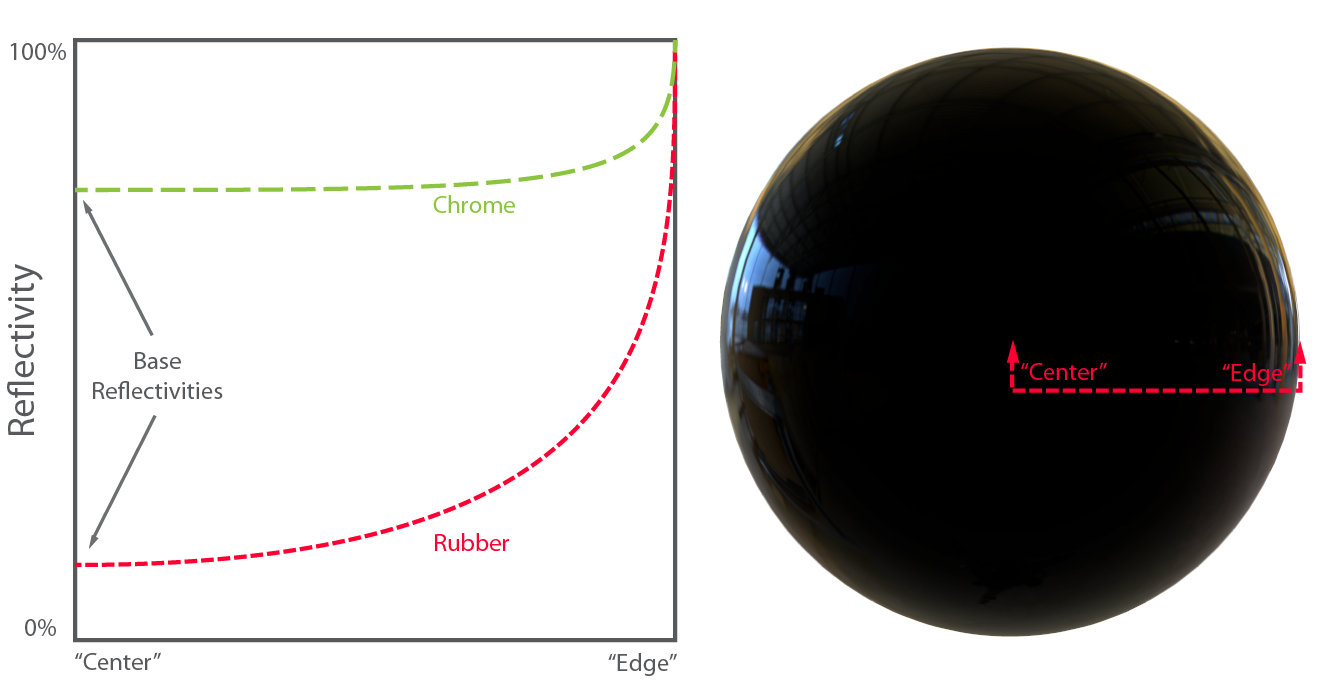Merging

In order to merge all this information into the final output color value, we need to calculate each part's contribution to it and add them together. The ambient factor is calculated as follows:

vec3 ambient = (kD * diffuse + specular) * ao_factor;

Being kD the refraction ratio, diffuse the irradiance multiplied by the albedo texture at that pixel, specular the prefiltered map value multiplied by the BRDF-LUT value, all based on roughness, and ao_factor the ambient occlusion value of the object at that pixel.

All that is left to do is to apply the high dynamic range (HDR) tonemapping, and gamma correction to the final output.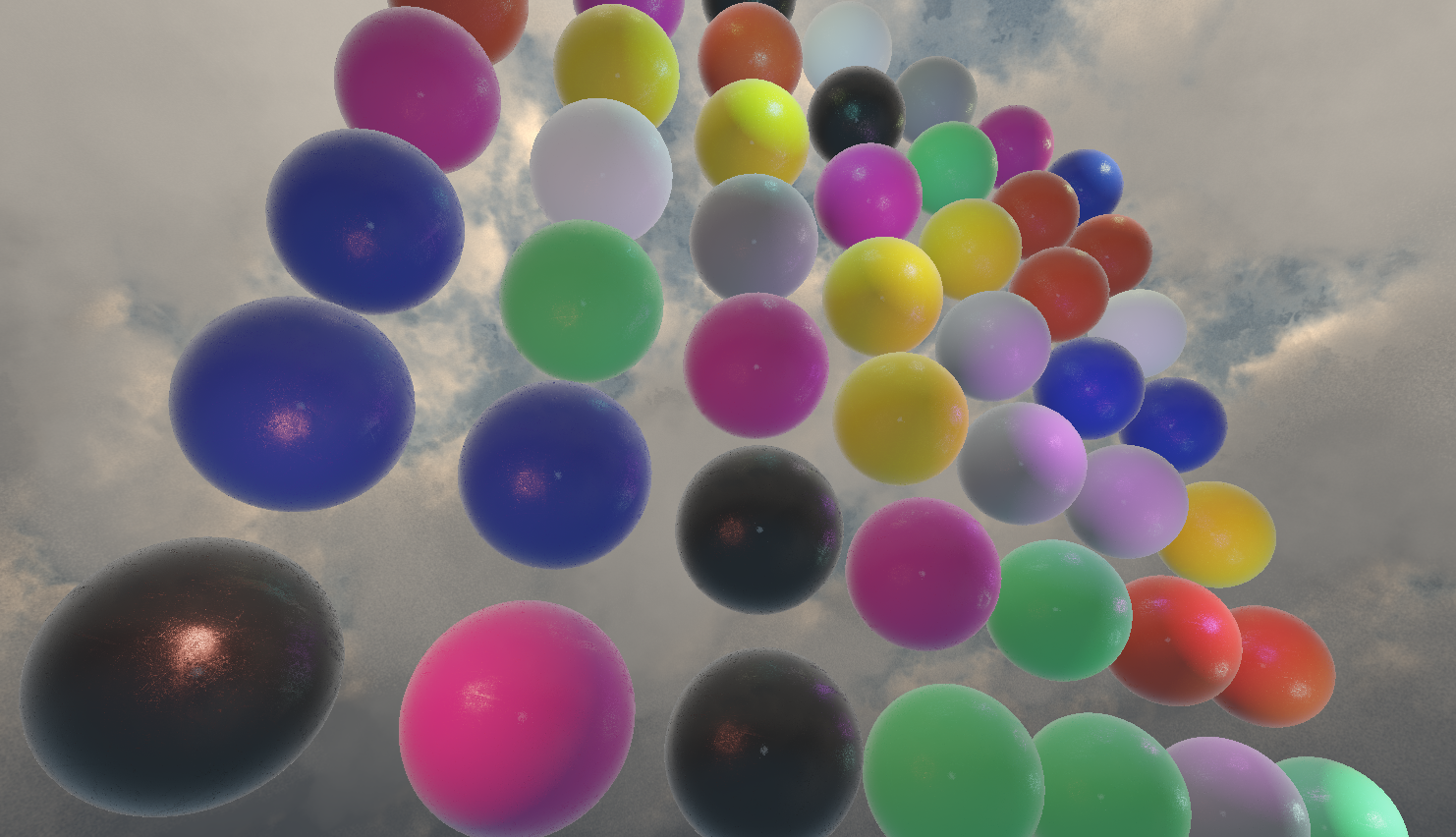Atmospheric Scattering

Even though this may seem like a completely unrelated topic, it really isn't. This technique simulates the color of the sky based on physically based light scattering equations. In order for this technique to be light-weight in performance, some simplification has to be done. In this case, the implementation assumes a single ray pointed to the viewers eye to simulate the light.Single Scattering

This form of ray tracing is called Single Scattering. It means that a single ray of light can be deflected many times, so that light can travel very complex paths when colliding with molecules before reaching the camera. There are two ways in which this molecules can affect our vision:

• Out-Scattering: happens when light is travelling towards the camera, and gets deflected by a collision with a particle. This is heavily affected by the distance that light has to travel. Distance makes the light to become progressively dimmer.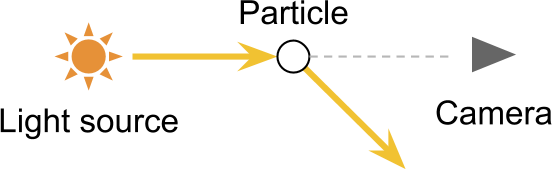• In-Scattering: happens when light is travelling in a direction, and gets deflected by a collision with a particle, which redirects it towards the camera. This allows us to see light sources that are not in the cameras direct light of sight, which causes halos around light sources.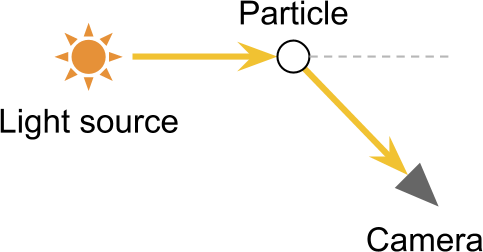Two of the most common forms of scattering in the atmosphere are Rayleigh scattering and Mie scattering.

Rayleigh Scattering

Rayleigh scattering is the reason why we see the sky blue from the surface of the earth; it is caused by rays colliding with small particules in the air, which causes shorter light wavelengths to collide more frequently with them, scattering blue and violet2 light along the sky, which bounces everywhere and makes the sky appear blue; however at sunset, the light has to travel through a lot more space so by the time it reaches our eye, all the blue and violet light is scattered away, leaving us only with the reddish light, making the sky appear orange and red.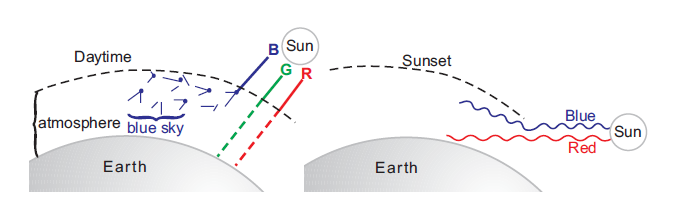2: Why is the sky blue and not violet? TL;DR, even though violet light wavelength is shorter than blue, the Sun sends many less violet rays than blue rays, so the sky appears tinted in blue!

Also, apart from this, the human eye has three types of cones to identify light color (red, green and blue). Blue cones, are most sensitive at blue wavelengths, but they drop sensitivity dramatically at violet wavelengths.

Mie Scattering

Mie scattering on the other side tends to scatter all wavelengths of light equally, as it refers to the collision with bigger particles (such as pollution and dust in the sky). It makes the sky look greyish and causes the sun to have a large white halo around it.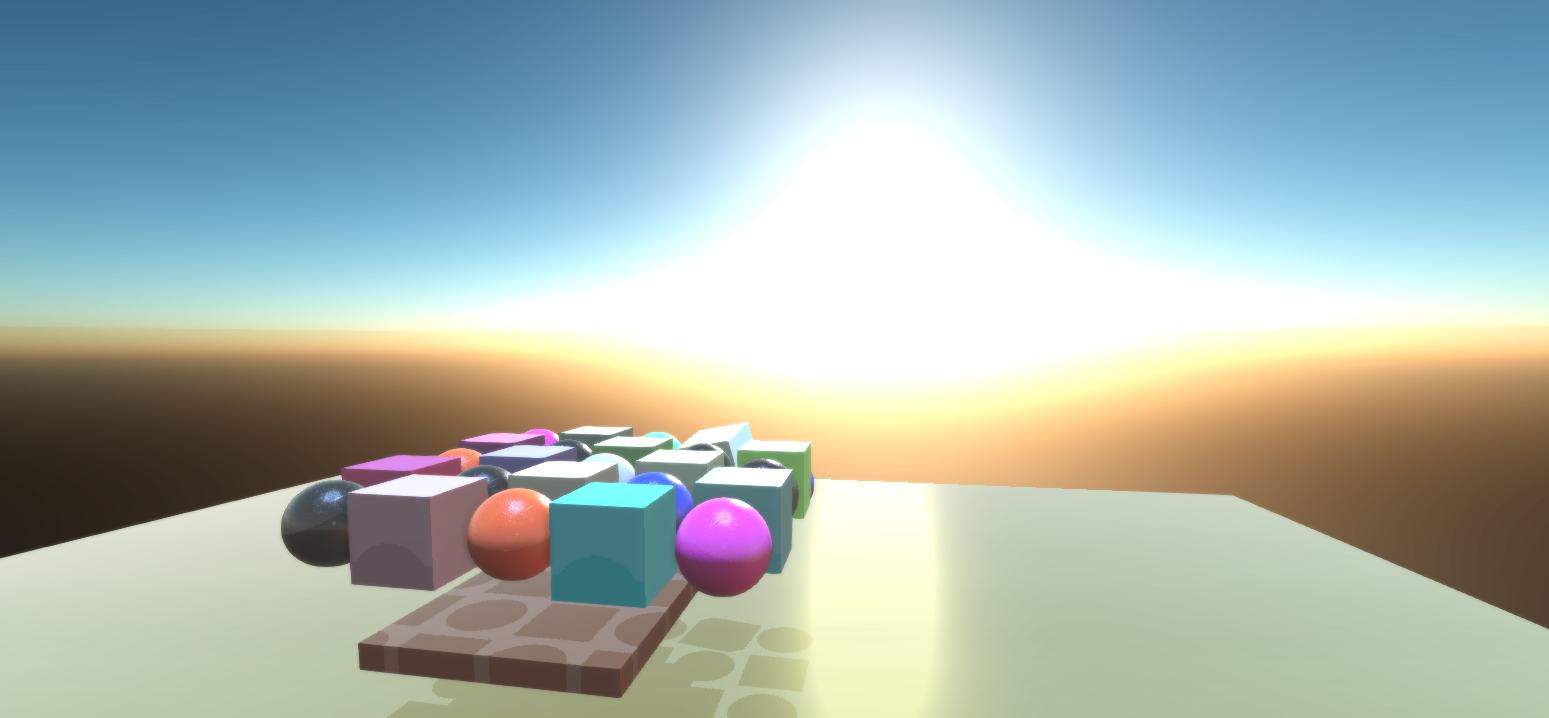Phase functions

The phase function describes how much light is scattered towards the diraction of the acamara based on the angle and a constant g that affects the symmetry of the scattering. This is one adaptation of the full function: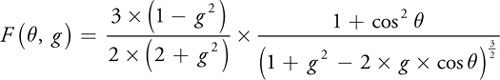However, for the sake of making this more lightweight, a huge level of simplification is applied to the function. The resulting equations used in the implementation are the following (for rayleigh and mie respectively):Finally, the result of this functions is computed per pixel and drawn onto a cubemap. In the engine you can modify some parameters to describe different colors in the sky. You can see me playing with some values in the demo video (second 1:25):